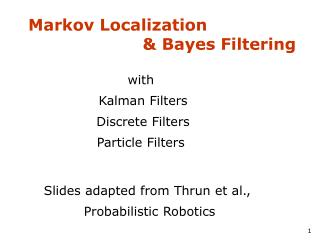DownloadDownload PresentationMarkov Localization & Bayes Filtering

# Markov Localization & Bayes Filtering

Download Presentation## Markov Localization & Bayes Filtering

- - - - - - - - - - - - - - - - - - - - - - - - - - - E N D - - - - - - - - - - - - - - - - - - - - - - - - - - -
##### Presentation Transcript

1. Markov Localization & Bayes Filtering with Kalman Filters Discrete Filters Particle Filters Slides adapted from Thrun et al., Probabilistic Robotics

2. Control Scheme for AutonomousMobile Robot

3. Control Scheme for AutonomousMobile Robot – the plan Thomas will cover generalized Bayesian filters for localization next week Mae sets up the background for him, today, by discussing motion and sensor models as well as robot control Mae then follows on Bayesian filters to do a specific example, underwater SLAM

4. Markov Localization The robot doesn’t know where it is. Thus, a reasonable initial believe of it’s position is a uniform distribution.

5. Markov Localization A sensor reading is made (USE SENSOR MODEL) indicating a door at certain locations (USE MAP). This sensor reading should be integrated with prior believe to update our believe (USE BAYES).

6. Markov Localization The robot is moving (USE MOTION MODEL) which adds noise.

7. Markov Localization A new sensor reading (USE SENSOR MODEL) indicates a door at certain locations (USE MAP). This sensor reading should be integrated with prior believe to update our believe (USE BAYES).

8. Markov Localization The robot is moving (USE MOTION MODEL) which adds noise. …

9. Bayes Formula

10. Bayes Rule with Background Knowledge

11. Normalization Algorithm:

12. Recursive Bayesian Updating Markov assumption: znis independent of z1,...,zn-1if we know x.

13. Putting oberservations and actions together: Bayes Filters • Given: • Stream of observations z and action data u: • Sensor modelP(z|x). • Action modelP(x|u,x’). • Prior probability of the system state P(x). • Wanted: • Estimate of the state X of a dynamical system. • The posterior of the state is also called Belief:

14. Graphical Representation and Markov Assumption Underlying Assumptions • Static world • Independent noise • Perfect model, no approximation errors

15. Bayes Markov Total prob. Markov Markov z = observation u = action x = state Bayes Filters

16. Prediction • Correction

17. Bayes Filter Algorithm • Algorithm Bayes_filter( Bel(x),d ): • h=0 • Ifd is a perceptual data item z then • For all x do • For all x do • Else ifd is an action data item uthen • For all x do • ReturnBel’(x)

18. Bayes Filters are Familiar! • Kalman filters • Particle filters • Hidden Markov models • Dynamic Bayesian networks • Partially Observable Markov Decision Processes (POMDPs)

19. Probabilistic Robotics Bayes Filter Implementations Gaussian filters

20. m Univariate -s s Gaussians Linear transform of Gaussians

21. Multivariate Gaussians • We stay in the “Gaussian world” as long as we start with Gaussians and perform only linear transformations.

22. Discrete Kalman Filter Estimates the state x of a discrete-time controlled process that is governed by the linear stochastic difference equation with a measurement

23. Linear Gaussian Systems: Initialization • Initial belief is normally distributed:

24. Linear Gaussian Systems: Dynamics • Dynamics are linear function of state and control plus additive noise:

25. Linear Gaussian Systems: Observations • Observations are linear function of state plus additive noise:

26. Kalman Filter Algorithm • Algorithm Kalman_filter( mt-1,St-1, ut, zt): • Prediction: • Correction: • Returnmt,St

27. Kalman Filter Summary • Highly efficient: Polynomial in measurement dimensionality k and state dimensionality n: O(k2.376 + n2) • Optimal for linear Gaussian systems! • Most robotics systems are nonlinear!

28. Nonlinear Dynamic Systems • Most realistic robotic problems involve nonlinear functions

29. Linearity Assumption Revisited

30. Non-linear Function

31. EKF Linearization (1)

32. EKF Linearization (2)

33. EKF Linearization (3)

34. EKF Linearization: First Order Taylor Series Expansion • Prediction: • Correction:

35. EKF Algorithm • Extended_Kalman_filter( mt-1,St-1, ut, zt): • Prediction: • Correction: • Returnmt,St

36. Localization “Using sensory information to locate the robot in its environment is the most fundamental problem to providing a mobile robot with autonomous capabilities.” [Cox ’91] • Given • Map of the environment. • Sequence of sensor measurements. • Wanted • Estimate of the robot’s position. • Problem classes • Position tracking • Global localization • Kidnapped robot problem (recovery)

37. Landmark-based Localization

38. EKF Summary • Highly efficient: Polynomial in measurement dimensionality k and state dimensionality n: O(k2.376 + n2) • Not optimal! • Can diverge if nonlinearities are large! • Works surprisingly well even when all assumptions are violated!

39. Kalman Filter-based System • [Arras et al. 98]: • Laser range-finder and vision • High precision (<1cm accuracy) [Courtesy of Kai Arras]

40. Multi-hypothesisTracking

41. Localization With MHT • Belief is represented by multiple hypotheses • Each hypothesis is tracked by a Kalman filter • Additional problems: • Data association: Which observation corresponds to which hypothesis? • Hypothesis management: When to add / delete hypotheses? • Huge body of literature on target tracking, motion correspondence etc.

42. MHT: Implemented System (2) Courtesy of P. Jensfelt and S. Kristensen

43. Probabilistic Robotics Bayes Filter Implementations Discrete filters

44. Piecewise Constant

45. Discrete Bayes Filter Algorithm • Algorithm Discrete_Bayes_filter( Bel(x),d ): • h=0 • Ifd is a perceptual data item z then • For all x do • For all x do • Else ifd is an action data item uthen • For all x do • ReturnBel’(x)

46. Grid-based Localization

47. Sonars and Occupancy Grid Map

48. Probabilistic Robotics Bayes Filter Implementations Particle filters

49. Sample-based Localization (sonar)